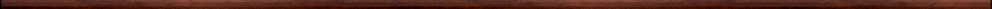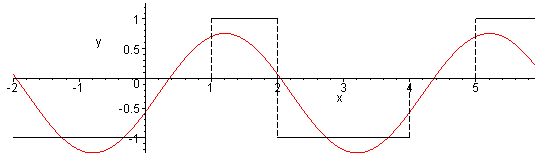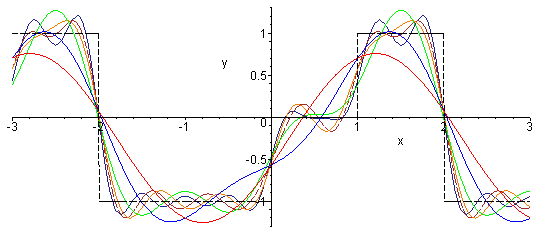Maple worksheets on Fourier seriesApproximation of functions:

They are all compatible with Classic Worksheet Maple 10.Preliminaries: even and odd functions, adding sine and cosine graphs - FRprelim.mws

• Even and odd functions.
• Examples of even functions.
• Examples of odd functions.
• Products and composites of even and odd functions.
• Integral formulas involving even and odd functions.
• Adding sine and cosine graphs.

An introduction to Fourier series - FRintro.mws

• Introductory examples
• Dot product of functions and orthogonal functions
• The Fourier series coefficients
• Examples

More period 2*Pi Fourier series examples - FRexamp.mws

• Convergence of a Fourier series
• Examples

Fourier series for general period 2*Pi functions - FRexamp2.mws

• Integrating a periodic function over a complete period.
• Fourier series coefficients for general periodic functions with period 2*Pi
• Examples

Fourier series for general periodic functions .. I - FRgen.mws

• Fourier of general periodic functions
• Fourier series .. summary
• Examples

Fourier series for general periodic functions .. 2 - FRgen2.mws

• Fourier series coefficients for general periodic functions
• Examples

A procedure for constructing Fourier series: FourierSeries - FRproc.mws

• A  procedure for constructing truncated Fourier series: FourierSeries
The procedure attempts to construct a general formula for the coefficients, but if this fails, individual coefficients are calculated separately. When integrals cannot be determined analytically, they are calculated numerically.

Fourier  sine and cosine series - FRsincos.mws

• Fourier sine series coefficients
• Examples
• Fourier cosine series coefficients
• Examples

Half-range Fourier series - FRhalf.mws

• Half-range sine and cosine series
• Examples

Fourier series of some functions with infinite discontinuities - FRmore.mws

Fourier series and Chebyshev series - FRcheb.mws

• Chebyshev polynomials
• Introductory example of a Chebyshev series
• More xamples

Further symmetry properties related to Fourier series - FRsym.mws

• Functions which are even or odd with respect to an interval (my terminology)
• Pseudo-even and pseudo-odd functions (my terminology again)
• The Fourier series of a pseudo-even function
• The Fourier series of a pseudo-odd function
• Simplification of the integral formulas for the Fourier series of pseudo-even and pseudo-odd functions
- associated "half-range" type series

DE's with a periodic forcing function and Fourier series - FRDE.mws

• An example of forced damped oscillations
•  Forced damped oscillations with a non-sinusoidal periodic forcing function

Fourier series for piecewise linear functions- FRpwlin.mws

• A procedure for constructing a piecewise linear function through given points: convert(.., pwlinear,x)
• Examples

The complex Fourier series - FRcomplex.mws

• Formulas for the coefficients in the complex Fourier series
• Orthogonality of the functions in the complex Fourier series
• The complex Fourier series via the orthogonality properties

Procedures for Fourier series and Fourier transforms - fourier.zipTop of page

Main index